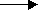# Energy

• Energy is the ability to do work.
• It is measured in Joules (J).

## Types of Energy

• Potential Energy is stored energy. There are 3 types of potential energy:
1. Chemical potential energy (in food, batteries and dynamite)
2. Elastic potential energy (in rubber bands and springs)
3. Gravitational potential energy (of objects some height above the ground)
• Kinetic Energy is the energy of moving objects.
• Heat or Thermal Energy
• Sound Energy
• Electrical Energy
• Nuclear Energy

## Gravitational Potential Energy

• Rule for Gravitational Potential Energy
 GPE (joules) = Mass (kg) × Gravitational Acceleration (9.8 m/s2) × Height (m) = m × g × h

• Example of the Gravitational Potential Energy Rule

Q. A ball with a mass of 2 kilograms is thrown to a height of 3 metres. What is its gravitational potential energy?
 A. GPE = m × g × h = 2 kg × 9.8 m/s2 × 3 m = 58.8 J (joules)

## Kinetic Energy

• Rule for Kinetic Energy
 KE (joules) = 1⁄2 Mass (kg) × Velocity2 (m/s) = 1⁄2 m × v2 = 1⁄2 mv2

• Example of Kinetic Energy Rule

Q. A ball of mass 2 kilograms is travelling at a speed of 10 m/s. What is its kinetic energy?
 A. KE = 1⁄2 m × v2 = 1⁄2 × 2 kg × 10 m/s × 10 m/s = 100 J

## Nuclear Energy

• Structure of Atoms - An atom is composed of a nucleus with protons (positive charge) and neutrons (no charge), surrounded by electrons (negative charge).
• Atomic Number - the number of protons in an atom
• Mass Number - the number of protons and neutrons together in an atom
• Isotopes - Isotopes are atoms which have different numbers of neutrons in the nucleus. For example, uranium has two main isotopes - Uranium-238 and Uranium-235. Uranium-238 is the more stable form and makes up about 99% of the world's uranium. Uranium-235 is the less stable form and is enriched (or concentrated) from the mined 0.7% to nuclear reactor grade of 3%.
• Nuclear Reactions - Nuclear reactions involve the formation of entirely new atoms. (Chemical reactions only rearrange existing atoms.) Nuclear reactions also give off radiation and energy, sometimes huge amounts of energy.
• Transmutation - Transmutation is the process of changing one element into another as occurs in nuclear reactions.
 RADIATION COMPONENT SPEED or VELOCITY PENETRATING POWER Alpha rays (α) Helium nuclei 5% of the speed of light Low Beta rays (β) Electrons Up to 90% of the speed of light Moderate Gamma rays (γ) Electromagnetic waves Speed of light High

• Two Types of Nuclear Reactions
1. Nuclear Fusion - involves the 'joining' of small nuclei (e.g. hydrogen) to make one larger nucleus (e.g. helium). This occurs in stars. Once the reaction is started, it creates sufficient energy to keep going.
2. Nuclear Fission - This nuclear reaction involves the 'splitting' of one large unstable nucleus into two smaller stable nuclei. A minimum amount (critical mass) of radioactive material such as Uranium-235 is needed to start the fission reaction. The extra neutrons released collide with more large nuclei splitting them in turn. This is called a chain reaction. If uncontrolled, the chain reaction creates a bomb. To control the fission reaction, control rods and moderators in nuclear reactors absorb the extra neutrons so that a 'melt-down' does not occur.
• Radioactive Decay - Different radioactive atoms decay or break down into smaller atoms at different rates. The half-life is the time taken for half of the original amount of radioactive isotope to decay.
 RADIOISOTOPE HALF-LIFE Polonium-215 0.0018 seconds Iron-59 46.3 days Carbon-14 5930 years Uranium-235 710 million years

## Law of Conservation of Energy

• Energy cannot be created nor destroyed in any chemical reaction. It can only be changed from one form to another.

## Energy Transformations

• Energy Transformations occur when the energy of an object changes or transforms from one type to a different type of energy.
• Examples of Energy Transformation
1. Light bulb
2. Electrical EnergyLight Energy + Heat Energy
3. Car Battery
4. Chemical Potential EnergyElectrical Energy

## Energy Transfers

• Energy Transfers occur when the same type of energy moves or transfers from one object to another object.
• Example of Energy Transfer - When water is heated in a saucepan, the heat energy of the stove transfers to heat energy of the saucepan to heat energy of the water.